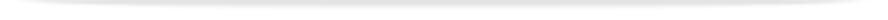units

ENG2005

Faculty of Engineeringprint version

This unit entry is for students who completed this unit in 2016 only. For students planning to study the unit, please refer to the unit indexes in the the current edition of the Handbook. If you have any queries contact the managing faculty for your course or area of study.

# ENG2005 - Advanced engineering mathematics

## 6 points, SCA Band 2, 0.125 EFTSL

Refer to the specific census and withdrawal dates for the semester(s) in which this unit is offered.

Faculty

Engineering

Coordinator(s)

A/Prof Michael Page

Offered

Not offered in 2016

### Synopsis

Advanced matrix algebra: mxn systems, linear independence, sparse matrices, simple tensors. Further ordinary differential equations: systems of ODEs, variation of parameters; boundary-value problems. Fourier series: Euler formulae, convergence, half-range series, solution of ODEs, spectra. Further multivariable calculus: change of variables and chain rule, polar coordinates, line integrals; vector fields; del, divergence, curl and Laplacian; surface and volume integrals; Gauss and Stokes theorems. Partial differential equations: simple PDEs, Laplace, heat and wave equations, superposition, separation of variables, polar coordinates. Advanced numerical methods: solution of linear systems, numerical solution of ODEs and simple PDEs, accuracy, efficiency and stability; discrete Fourier transforms, introduction to PS and FE methods.

### Outcomes

Upon successful completion of this unit, students will be able to:

1. use essential concepts related to mxn linear systems, including linear independence and basis, and demonstrate a broad appreciation of tensors
2. solve systems of simple ordinary differential equations, establish and use their eigenvalues, solve simple second-order boundary-value problems
3. represent a periodic function with a Fourier series, determine their convergence, calculate even and odd series, and apply these to solving simple periodic systems
4. perform change of variables for multivariable functions with the chain rule, use polar coordinates, represent 2D and 3D curves parametrically and solve line integrals on these curves
5. manipulate and evaluate double and triple integrals in Cartesian, cylindrical and spherical coordinates
6. calculate the gradient, divergence and curl vector operations, and apply these in the evaluation of surface and volume integrals through the Gauss and Stokes theorems
7. solve elementary partial differential equations, apply boundary and initial conditions as appropriate, and use the method of separation of variables with the wave equation, heat equation and Laplace's equation
8. appreciate key issues related to the numerical solution of full and sparse linear systems
9. apply a range of suitable techniques for the numerical solution of ODEs, including using discrete Fourier transforms, PS and FE methods
10. use a range of suitable simple numerical techniques for the solution of PDEs and appreciate their advantages and disadvantages
11. use MATLAB and other appropriate software to assist in understanding these mathematical techniques

express and explain mathematical techniques and arguments clearly in words.

### Assessment

Continuous assessment: 30%
Examination (3 hours): 70%

Students are required to achieve at least 45% in the total continuous assessment component and at least 45% in the final examination component and an overall mark of 50% to achieve a pass grade in the unit. Students failing to achieve this requirement will be given a maximum of 45% in the unit.

Four 1-hour lectures (or equivalent), one 2-hour practice class and 6 hours of private study per week